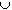#Interactive Real Analysis

Next | Previous | Glossary | Map

## 5.1. Open and Closed Sets

### Examples 5.1.6(b):

Find the boundary, interior, isolated and accumulation points, if any, for the set
• {1, 1/2, 1/3, ... }{0}
• The boundary of the set is the set itself, because any neighborhood of every point contains that point itself and some irrational points. Therefore, any neighborhood of every point contains points from within and from without the set, i.e. every point of the set is a boundary point.
• The interior of this set is empty, because if x is any point in that set, then any neighborhood of x contains at least one irrational point that is not part of the set. Therefore, this neighborhood is not contain in the set.
• Every point except 0 is an isolated point. First, it is easy to find a small enough neighborhood for any point of the form x = 1/n that does not contain any point from the set but x = 1/n. Therefore, every point x = 1/n is isolated. On the other hand, if (-a, a) is any small neighborhood of 0, then if n is large enough we have that 1/n < a. But then the point 1/n which is different from 0, is also part of the neighborhood, and hence 0 is not isolated.
• The point 0 is the only accumulation point of the set. It is clear that no point of the form x = 1/n is an accumulation point, and it is also clear that 0 is an accumulation point. Simply look at the previous example, and recall the definition of accumulation points and their related points.
Next | Previous | Glossary | Map